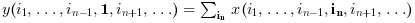Scilab Home page | Wiki | Bug tracker | Forge | Mailing list archives | ATOMS | File exchange
Scilab 6.0.2
Change language to: Français - Português - 日本語 - Русский

sum

sum of array elements

Syntax

y=sum(x)
y=sum(x,orientation)
y=sum(x,outtype)
y=sum(x,orientation,outtype)

Arguments

x

an array of reals, complex, booleans, polynomials or rational fractions.

orientation

it can be either

• a string with possible values "*", "r", "c" or "m"

• a number with positive integer value

outtype

a string with possible values "native" or "double".

y

scalar or array

Description

For an array x, y=sum(x) returns in the scalar y the sum of all the elements of x.

y=sum(x,orientation) returns in y the sum of x along the dimension given by orientation:

• if orientation is equal to 1 or "r" thenor• if orientation is equal to 2 or "c" then:or• if orientation is equal to n then• y=sum(x,"*") is equivalent to y=sum(x)

• y=sum(x,"m") is equivalent to y=sum(x,orientation) where orientation is the index of the first dimension of x that is greater than 1.

The outtype argument rules the way the summation is done:

• For arrays of floats, of polynomials, of rational fractions, the evaluation is always done using floating points computations. The "double" or "native" options are equivalent.

• For arrays of integers,

if outtype="native" the evaluation is done using integer computations (modulo 2^b, where b is the number of bits used),

if outtype="double" the evaluation is done using floating point computations.

The default value is outtype="native".

• For arrays of booleans,

if outtype="native" the evaluation is done using boolean computations ( + is replaced by |),

if outtype="double" the evaluation is done using floating point computations (%t values are replaced by 1 and %f values by 0).

The default value is outtype="double".This function applies with identical rules to sparse matrices

Examples

A=[1,2;3,4];
sum(A)
sum(A,1)

I=uint8([2 95 103;254 9 0])
sum(I) //native evaluation
sum(I,"double")
sum(I,2,"double")

s=poly(0,"s");
P=[s,%i+s;s^2,1];
sum(P),
sum(P,2)

B=[%t %t %f %f];
sum(B) //evaluation in float
sum(B,"native") //similar to or(B)